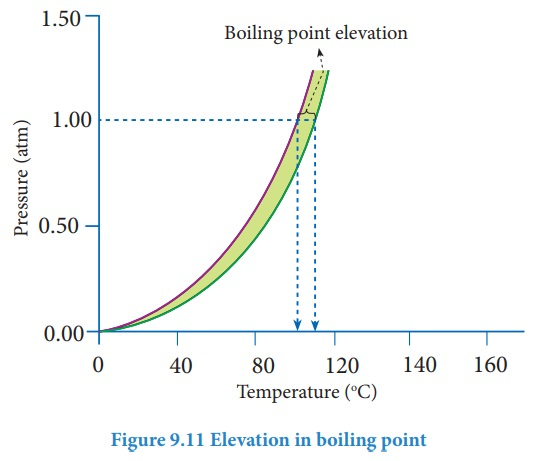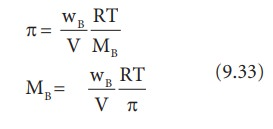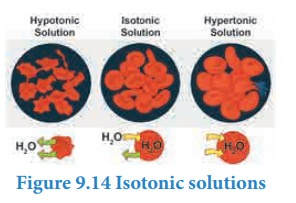Home | | Chemistry 11th std | Colligative properties

# Colligative properties

Pure water is tasteless. When you add sugar it becomes sweet, while addition of salt makes it salty.

Colligative properties

Pure water is tasteless. When you add sugar it becomes sweet, while addition of salt makes it salty. It implies that the properties of a solution depend on the nature of solute particles present in the solution. However, for an ideal dilute solution, the properties, namely, relative lowering of vapour pressure, elevation of boiling point, depression in freezing point and osmotic pressure do not depend on the chemical nature of the solute but depends only on the number of solute particles (ions/molecules) present in the solution. These four properties are known as colligative properties. Though the magnitude of these properties are small, they have plenty of practical applications. For example the osmotic pressure is important for some vital biological systems.

## Relative lowering of vapour pressure (╬öP)

The vapour pressure of a solution containing a nonvolatile, non-electrolyte solute is always lower than the vapour pressure of the pure solvent. Consider a closed system in which a pure solvent is in equilibrium with its vapour. At equilibrium the molar Gibbs free energies of solvent in the liquid and gaseous phase are equal (╬öG = 0). When a solute is added to this solvent, the dissolution takes place and its free energy (G) decreases due to increase in entropy. In order to maintain the equilibrium, the free energy of the vapour phase must also decrease. At a given temperature, the only way to lower the free energy of the vapour is to reduce its pressure. Thus the vapour pressure of the solution must decrease to maintain the equilibrium.

We know that from the Raoult's law the relative lowering of the vapour pressure is equal to the mole fraction of the solute (equation 9.10)From the above equation,it is clear that the relative lowering of vapour pressure depends only on the mole fraction of the solute (xB) and is independent of its nature. Therefore, relative lowering of vapour pressure is a colligative property.

## Determination of molar mass weights from relative lowering of vapour pressure

The measurement of relative lowering of vapour pressure can be used to determine the molar mass of a nonvolatile solute. For this purpose, a known mass of the solute is dissolved in a known quantity of solvent. The relative lowering of vapour pressure is measured experimentally.

According to RaoultŌĆÖs law the relative lowering of vapor pressure is,Let w A and wB be the weights of the solvent and solute respectively and their corresponding molar masses are MA and MB, then the mole fraction of the solute xB isHere, nA & nB are the moles of the solvent and the solute respectively. For dilute solutions nA>>nB. Hence nA +nB Ōēł nA. NowNumber of moles of solvent and the solute are,From the equation (9.35) the molar mass of the solute (MB) can be calculated using the known the values of wA, wB, MA and the measured relative lowering of vapour pressure.

### Example Problem 3:

An aqueous solution of 2 % nonvolatile solute exerts a pressure of 1.004 bar at the boiling point of the solvent. What is the molar mass of the solute when PA┬░ is 1.013 bar?In a 2 % solution weight of the solute is 2 g and solvent is 98 g

╬öP = PA┬░ ŌĆō Psolution= 1.013 -1.004 bar = 0.009 barMB = 2 x 18 x 1.013/(98 x 0.009)

= 41.3 g mol-1

### Elevation of boiling point

Boiling point is an important physical property of a liquid. The boiling point of a liquid is the temperature at which its vapour pressure becomes equal to the atmospheric pressure (1 atm). When a nonvolatile solute is added to a pure solvent at its boiling point, the vapour pressure of the solution is lowered below 1 atm. To bring the vapour pressure again to 1 atm, the temperature of the solution has to be increased. As a result,the solution boils at a higher temperature (Tb) than the boiling point of the pure solvent (Tb┬░). This increase in the boiling point is known as elevation of boiling point. A plot of vapour pressure versus temperature for water and an aqueous solution is given belowThe vapour pressure of the solution increases with increase in temperature as shown in the above figure. The variation of vapour pressure with respect to temperature for pure water is given by the violet coloured curve. At 100 Ōü░C the vapour pressure of water is equal to 1 atm. Hence the boiling point of water is 100 Ōü░C (Tb┬░). When a solute is added to water, the vapour pressure of the resultant solution is lowered. The variation of vapour pressure with respect to temperature for the solution is given by green curve. From the graph, it evident the vapour pressure of the solution is equal to 1 atm pressure at the temperature Tb which is greater than Tb┬░. The difference between these two temperatures (Tb-Tb┬░) gives the elevation of boiling point.

The elevation of boiling point (╬öTb)= Tb - Tb┬░

The elevation of boiling point is directly proportional to the concentration of the solute particles.

╬öTb ╬▒ m (9.23)

m is the concentration of solution expressed in molality.

╬öTb = Kb m (9.24)

Where

Kb = molal boiling point elevation constant or Ebullioscopic constant.

If m=1, then ╬öTb=Kb;

Hence, the Kb is equal to the elevation in boiling point for 1 molal solution. Kb is calculated by the following expressionProblem:

0.75 g of an unknown substance is dissolved in 200 g water. If the elevation of boiling point is 0.15 K and molal elevation constant is 7.5 K Kg mol-1 then, calculate the molar mass of unknown substance

╬öTb  = Kb m

= Kb x W2 x 1000 / M2 x W1

M2 = Kb x W2 x 1000 / ╬öTb x W1

= 7.5 x 0.75 x 1000 / 0.15 x 200

= 187.5 g mol-1

### Depression in freezing point

Freezing point of a substance is another important physical property like boiling point. Freezing point is defined as ŌĆ£the temperature at which the solid and the liquid states of the substance have the same vapour pressureŌĆØ. At freezing point, the solid and liquid phases of the substance are in equilibrium. For example, the freezing point of water is 0 ╦ÜC. At this temperature the ice and the water are in equilibrium. When a nonvolatile solute is added to water at its freezing point, the freezing point of the solution is lowered from 0 ╦ÜC. The lowering of the freezing point of the solvent when a solute is added is called depression in freezing point (╬öTf).From the above graph, we infer that the freezing point (Tfo) is 0 ╦ÜC as the vapour pressure at this temperature is 1 atm (atmospheric pressure). The vapour pressure versus temperature curve for the solution indicates that the freezing point (Tf) is lower than the 0 ╦ÜC. The depression in freezing temperature (╬öTf) can be expressed as,

╬öTf = Tfo- Tf

The experimental results show that the depression in freezing point is directly proportional to the molal concentration of the solute particles.

Hence,

╬öTf ╬▒ m

╬öTf=Kf  m (9.25)

Here, ŌĆśmŌĆÖ = is the molality of the solution

Kf = molal freezing point depression constant or cryoscopic constant.

If m=1 then ╬öTf =Kf

The Kf is equal to the depression in freezing point for 1 molal solution

Table 9.4 Molal freezing point depression constant for some solvents### Determination of molar mass of solute from depression in freezing point

If the solution is prepared by dissolving wB g of solute in wA g of solvent, then the molality is,Example Problem ŌĆō 5

Ethylene glycol (C2H6O2) can be at used as an antifreeze in the radiator of a car. Calculate the temperature when ice will begin to separate from a mixture with 20 mass percent of glycol in water used in the car radiator. Kf for water = 1.86 K Kg mol-1 and molar mass of ethylene glycol is 62 g mol-1.

Weight of solute (W2) = 20 mass percent of solution means 20 g of ethylene glycol

Weight of solvent (water) W1 = 100 -20 = 80 g

╬öTf = Kfm= 7.5 K

The temperature at which the ice will begin to separate is the freezing of water after the addition of solute i.e 7.5 K lower than the normal freezing point of water (273-7.5K) = 265.5 K

## Osmosis and osmotic pressure

Many biological processes depend on osmosis, which is a spontaneous process by which the solvent molecules pass through a semi permeable membrane from a solution of lower concentration to a solution of higher concentration. The name osmosis is derived from the Greek word ŌĆśosmosŌĆÖ which means ŌĆśto pushŌĆÖ. It is also important to know that the semipermeable membrane selectively allows certain molecules in the solution to pass through it but not others.Let us consider a simple apparatus as shown in the above figure. A semipermeable membrane separates a chamber into two compartments. Water (pure solvent) is added to the first compartment and the aqueous NaCl (solution) is added to the second compartment such that the liquid levels on the both sides are equal. Since there is a difference in concentration between the liquids present in the two compartments, the water molecules move from first compartment to second compartment through the semipermeable membrane. The membrane allows only the water molecules to pass through it in either direction but not allows the NaCl. The net flow of water is into the sodium chloride solution and hence increases its volume. This decreases its concentration and also creates a pressure difference between the compartments. This pressure difference, push some of the water molecules back to the solvent side through the semipermeable membrane until an equilibrium is established. At the equilibrium, the rate of movement of solvent molecules on both directions are equal. The pressure difference at the equilibrium is called osmotic pressure (ŽĆ). Thus, osmotic pressure can be defined as ŌĆ£the pressure that must be applied to the solution to stop the influx of the solvent (to stop osmosis) through the semipermeable membraneŌĆØ

vanŌĆÖt Hoff found out that for dilute solutions, the osmotic pressure is directly proportional to the molar concentration of the solute and the temperature of the solution. He proposed the following equation to calculate osmotic pressure which is now called as vanŌĆÖt Hoff equation.

ŽĆ=cRT ...........9.31

Here,

c = Concentration of the solution in molarity

T = Temperature

R = Gas constant

### Determination of molar mass from osmotic pressure

According to vanŌĆÖt Hoff equation

ŽĆ=cRT

c= n / V

Here, n= number of moles of solute dissolved in ŌĆśVŌĆÖ litre of the solution.

Therefore, ŽĆ = n/V . RT or

ŽĆV=nRT (9.32)

If the solution is prepared by dissolving wB g of nonvolatile solute in wA g of solvent, then the number of moles ŌĆśnŌĆÖ is,

n= wB/MB

Since,MB = molar mass of the solute

Substituting the ŌĆśnŌĆÖ in 9.32, we get,From the equation 9.33, molar mass of the solute can be calculated.

### Significances of osmotic pressure over other colligative propertiesUnlike elevation of boiling point (for 1 molal solution the elevation in boiling point is 0.512 ╦ÜC for water) and the depression in freezing point (for 1 molal solution the depression in freezing point is 1.86 ╦ÜC for water), the magnitude of osmotic pressure is large.

The osmotic pressure can be measured at room temperature enables to determine the molecular mass of biomolecules which are unstable at higher temperatures.

Even for a very dilute solution, the osmotic pressure is large.

## Isotonic solutions

Two solutions having same osmotic pressure at a given temperature are called isotonic solutions. When such solutions are separated by a semipermeable membrane, solvent flow between one to the other on either direction is same, i.e. the net solvent flow between the two isotonic solutions is zero.The osmotic pressure of the blood cells is approximately equal to 7 atm at 37╦ÜC. The intravenous injections should have same osmotic pressure as that of the blood (isotonic with blood). If the Intravenous solutions are too dilute that is hypotonic, the solvent from outside of the cells will flow into the cell to normalise the osmotic pressure and this process which is called hemolysis, causes the cells to burst. On the other hand, if the solution is too concentrated, that is hypertonic, the solvent molecules will flow out of the cells, which causes the cells to shrink and die. For this reason, the Intravenous fluids are prepared such they are isotonic to blood (0.9 % mass/ volume sodium chloride solution).

Tags : Solutions | Chemistry , 11th Chemistry : UNIT 9 : Solutions
Study Material, Lecturing Notes, Assignment, Reference, Wiki description explanation, brief detail
11th Chemistry : UNIT 9 : Solutions : Colligative properties | Solutions | Chemistry